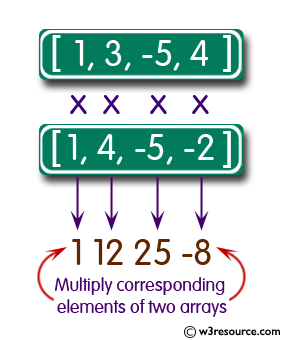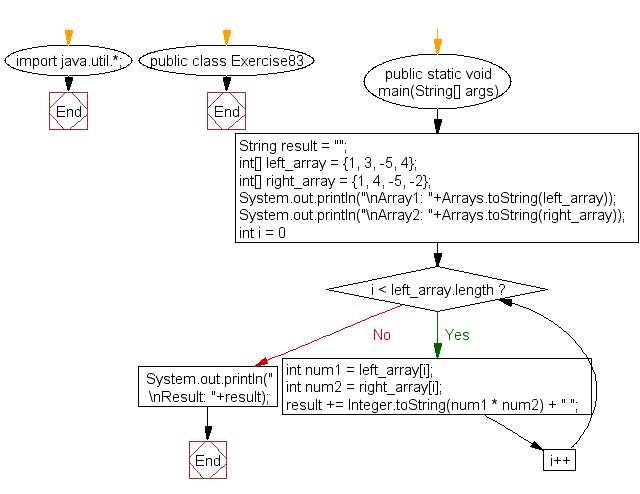﻿ Java: Multiply corresponding elements of two arrays# Java Exercises: Multiply corresponding elements of two arrays of integers

## Java Basic: Exercise-83 with Solution

Write a Java program to multiply corresponding elements of two arrays of integers.

Sample Solution:

Java Code:

``````import java.util.*;
public class Exercise83 {
public static void main(String[] args){
String result = "";
int[] left_array = {1, 3, -5, 4};
int[] right_array = {1, 4, -5, -2};
System.out.println("\nArray1: "+Arrays.toString(left_array));
System.out.println("\nArray2: "+Arrays.toString(right_array));
for (int i = 0; i < left_array.length; i++) {
int num1 = left_array[i];
int num2 = right_array[i];
result += Integer.toString(num1 * num2) + " ";
}
System.out.println("\nResult: "+result);
}
}
```
```

Sample Output:

```Array1: [1, 3, -5, 4]

Array2: [1, 4, -5, -2]

Result: 1 12 25 -8
```

Pictorial Presentation:Flowchart:Java Code Editor:

What is the difficulty level of this exercise?

Test your Programming skills with w3resource's quiz.

﻿

## Java: Tips of the Day

countOccurrences

Counts the occurrences of a value in an array.

Use Arrays.stream().filter().count() to count total number of values that equals the specified value.

```public static long countOccurrences(int[] numbers, int value) {
return Arrays.stream(numbers)
.filter(number -> number == value)
.count();
}
```

Ref: https://bit.ly/3kCAgLb